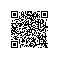# 《从问题到程序：用Python学编程和计算》——3.4　定义函数

### 3.4　定义函数

3.4.1　为什么定义函数

c1 = cube(x)
c2 = cube(y)


c1 = x * x * x
c2 = y * y * y


• 作用1：把适当的代码片段包装为函数定义，有可能缩短程序。这是最直接的功利性原因。如果一个函数的定义（函数体）比较长，而且在程序里多处调用，这种效果就会很明显。很长的函数定义只需要写一次，函数调用的代码非常简单。
• 作用2：把一种有用的计算功能集中在一个地方描述，用一个函数定义包装起来，这样做可以带来很多实际利益。例如，如果发现完成这部分工作的代码有错，则只需要在一个地方修正（只要函数的调用形式不变）。实际上，写好的程序需要修改是编程中经常要做的工作，并不只是发现错误之后的工作。例如，将来可能发现完成这一计算的新方法，或者计算的环境或者要求发生了变化，程序要增加功能，等等。要在大程序里的许多地方做同样修改，是一件非常耗时的工作，而且很容易弄错。有了函数，就可以省时、省力，更容易正确地完成这一工作。
• 作用3：定义函数是定义新的编程概念，扩充所用的语言。例如，Python语言里原来没有立方的概念，定义了计算立方的cube函数，就是给语言增加了这个新概念。在此之后写程序，讨论程序，或者思考程序的问题时，就都能使用这个新概念了。与此相对，无论在程序里写过多少次x x x，也没引进新的编程概念。
编程语言（例如Python）是通用的，只能提供一批在许多程序都可能用到的基本概念和基本结构。实际需要的每个程序都是具体的，处理具体领域的问题。只使用语言的基本机制编程序，在解决复杂的问题时，程序变长的同时也会变得越来越不清晰，越来越难理解，以至于变得无法把握，不能正确写出。

• 作用4：反过来看，函数也是一种功能分解机制，可以用于分解复杂问题，分解程序的复杂性。在程序开发过程中，可以把要开发的复杂功能分解为一些概念清晰、功能较为简单的待开发函数。如果分解产生的问题仍然比较复杂，可以考虑做进一步分解。这样做也分解了程序的实现结构，分解了实现的困难。
• 作用5：以函数作为程序单位，可用于划分开发工作。解决复杂问题的程序通常需要多人参与，为此需要把编程工作分解为一些相对独立的部分。函数作为一种很自然的独立程序单位，适合分配给不同的人去开发。
• 作用6：无论是不是多人参与的程序工作，上述局部性都很有意义。一个需求清晰的独立单元可以作为一个独立的工作对象，独立开发，独立地检查和调试。适当控制规模，又具有独立性，出现错误也比较容易发现和更正。即使是一个人做程序，函数分解也可以作为划分自己工作阶段和步骤的手段。
• 作用7：由于函数具有一定的独立性和通用性，设计良好的函数，其使用有可能超越当前开发的程序。如果一个函数提供了某种具有普遍意义的通用功能，开发者可以对其进行认真的设计和检查，并设法调整实现方式使其具有较高的效率。这样的工作成果（函数）不仅可以用于当前程序，还可能用于下一个程序或者别人的程序。这种工作方式称为程序（或程序部分）的重用。Python的标准库和其他库就是这方面的典范。例如，数学函数库就是开发者做出的一组函数，经过严格检查和优化，能保证其功能有效性和计算效率，供所有用Python语言写程序的人共享。
函数和函数分解还有很多可能的作用。此外，除了函数可以作为有用的程序模块外，Python还提供了另外一些可以作为程序模块的结构，有关情况将在后面介绍。

3.4.2　学习定义函数

• 确定函数的功能。这是第一位的问题，也是定义函数的第一步。
• 选定函数的参数。选参数是确定函数的功能抽象，把对具体数据的计算抽象为相对于一组参数的通用计算。究竟应该把计算中涉及的哪些数据抽取出来作为参数，把函数定义为针对它们的通用计算过程，这个问题需要认真考虑。
• 为函数命名。选择名字的问题相对简单，但为函数选择一个合适的名字，却是人们在编程实践中非常重视的问题，因为“名不正则言不顺”。
• 选择适当的实现方法（算法），实现函数体。在定义函数的工作中，这个部分的工作量最大。但如果其他部分做的很好，这里的问题很具体：一是选择适当的计算方法，即算法，二是正确实现所选的算法（编程）。
最后一个问题虽然很重要，但却是一般编程都需要考虑和处理的问题，不是仅与定义函数有关的特殊问题，因此不是本节主题。下面主要关注前两个问题。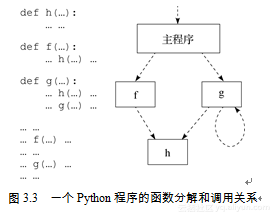• 程序中重复出现的相同或相似片段。可以考虑从中抽取出共性的东西定义为函数。这样做不但可能缩短程序代码，也将提高程序的可读性和易修改性。
• 程序中具有逻辑独立性的片段。即使这种片段只出现一次，也可以考虑把它们定义为独立的函数，在需要执行的位置写函数调用。这种做法的主要作用是分解程序复杂性，使之更容易理解和把握。例如，许多程序可以分解为三个主要工作阶段：正式工作前的准备阶段，主要工作阶段（这里常有复杂的循环等），完成前的结束处理。把程序分解为相应三部分，设计好它们之间的信息联系方式后，就可以用独立的函数分别实现了。显然，与整个程序相比，各部分的复杂性都降低了。
很难说什么是一个程序的最佳分解。实际上，对一个程序可能有多种可行分解方案，寻找比较合理或有效的分解方式是需要学习的东西。熟悉程序设计的人们提出的一条经验准则是：如果一段计算可以定义为函数，那么就应该把它定义为函数。https://yqfile.alicdn.com/b3f0b84f28832a24533bd992aafd7beda1c98c98.png" >

def line(begin, end):... ...
def ends(first, second): ... ...


def line(begin, end, ch): ... ...
def ends(first, second, ch): ... ...


for i in range(10):
print(ends(10 – i, 10 + i))
print(line(0, 20))


def line(begin, end):
s = ""
for i in range(0, begin):
s += " "
for i in range(begin, end + 1):
s += "*"

return s

def ends(first, second):
s = ""
for i in range(0, first):
s += " "
s += "*"
if first < second:
for i in range(first + 1, second):
s += " "
s += "*"

return s


def rect(begin, len, high): ... ...
def rect_fill(begin, len, high): ... ...


def rect(begin, len, high, fill): ... ...


def symbs(ch, n): ... ...


3.4.3　函数：两种观点及其联系https://yqfile.alicdn.com/3ac5626ff32ec84d4bf84705be4a31ae888a6f8a.png" >

• 要求某个参数是整数（例如阶乘函数），或者是数值（整数或浮点数，例如求绝对值的函数），或者是字符串等。这是对实际参数的类型要求。
• 要求某参数的值必须为正，这是对实际参数的值要求。

def triangle(a, b, c):
if a > 0 and b > 0 and c > 0 and \
a + b > c and b + c > a and a + c > b:
s = (a + b + c)/2
return (s * (s – a) * (s – b) * (s – c))**0.5
else:
return float("nan")


def triangle(a, b, c):
if (ininstance(a, int) or isinstance(a, float)) and \
(ininstance(b, int) or isinstance(b, float)) and \
(ininstance(c, int) or isinstance(c, float)) and \
a > 0 and b > 0 and c > 0 and \
a + b > c and b + c > a and a + c > b:
s = (a + b + c)/2
return (s * (s – a) * (s – b) * (s – c))**0.5
else:
return float("nan")


>>> print(abs.__doc__)
abs(number) -> number

Return the absolute value of the argument.


>>> abs("abc")
Traceback (most recent call last):
File "<pyshell#15>", line 1, in <module>
abs("abc")
TypeError: bad operand type for abs(): 'str'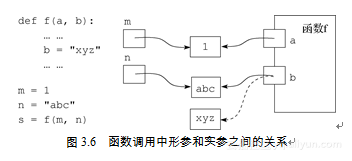1）保证只用符合函数实际需要的实参去调用。这就要求在每个函数调用前检查实参的值，满足条件时才调用函数。这件事可以用if语句做。但是这里也有麻烦：没有通过检查的情况怎么办？还是处理错误数据的问题，逃不掉。
2）在调用函数之后检查结果，确定返回值正确后再使用，不正确的情况另行处理。

def ratio(x1, x2, y1, y2):
return (x2 – x1) // (y2 – y1)


assert 条件
assert 条件, 表达式


def fact(n):
assert isinstance(n, int) and n >= 0
prod = 1
for k in range(2, n+1):
prod = prod * k
return prod


>>> fact(-2)
Traceback (most recent call last):
File "<pyshell#4>", line 1, in <module>
fact(-2)
File "D:/Progs/pyptop-02/fact-assert.py", line 4, in fact
assert n >= 0
AssertionError


def fact(n):
assert isinstance(n, int) and n >= 0, "Argument is " + str(n)
prod = 1
for k in range(2, n+1):
prod = prod * k
return prod


>>> fact(-3)
Traceback (most recent call last):
File "<pyshell#3>", line 1, in <module>
fact(-3)
File "D:\MyBooks\ptop-Python\Progs\basic.py", line 98, in fact
assert isinstance(n, int) and n >= 0, "Argument is " + str(n)
AssertionError: Argument is -3


• 使程序可能出现的错误得以及早报告，而不是让错误数据流入后续计算步骤，直到某个无法执行的操作报告一个难以理解的错误，或造成程序不声不响的错误行为（例如算出完全无意义的值，或者造成其他破坏）。
• 适当描述的断言有助于错误定位和更正。特别的，如果采用第二种形式提供更多现场信息，将使编程序的人更容易确定错误的原因。
• 断言形成了一种有实际效果的嵌入式程序文档，明确描述程序运行中必须满足的条件，有助于程序阅读和理解（包括开发者和其他人）。
3.4.4　通用和专用的方法

def cbrt(x):
x = float(x)  # 能转换也说明 x 是合理的参数
sign = -1 if x < 0.0 else 1
x = abs(x)
test, root = 0.0, 0.0

while test**3 <= x:
if abs(test**3 - x) < abs(root**3 - x):
root = test
test += 0.0001

return sign * root


>>> cbrt(2)**3
1.9956169789998675
>>> cbrt(20)**3
19.990770343995848
>>> cbrt(200)**3
199.9963601920295
>>> cbrt(2000)**3
1999.899757798265


def cbrt(x):
y = abs(x)
a, b = 0.0, y
while True:
m = (a + b)/2
if abs(m**3 - y) < 0.001:
return -m if x < 0.0 else m
if m**3 > y:
b = m
else:
a = m


>>> cbrt(200.0)**3
199.9990560136106
>>> cbrt(20000.0)**3
20000.000561170757


if y >= 1:
a, b = 1.0, y
else:
a, b = y, 1.0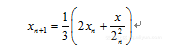def cbrt(x):
if x == 0.0:
return 0.0
x1 = x
while True:
x1 = (2.0 * x1 + x / x1 / x1) / 3
if abs(x1**3 - x) < 0.001:
return x1https://yqfile.alicdn.com/c83e07ddd4439236e883ea2be5fbecf05db76fce.png" >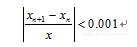def cbrt(x):
if x == 0.0:
return 0.0
x1 = x
while True:
x2 = (2.0 * x1 + x / x1 / x1) / 3
if abs((x2 - x1) / x1) < 0.001:
return x2
x1 = x2


>>> cbrt(200.0)**3
200.00001782197174
>>> cbrt(0.01)**3
0.010000000022781627